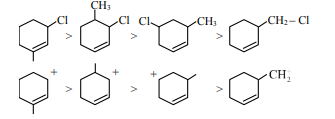# The correct order of reactivity of the given chlorides with acetate in acetic acid is :`
Question:

The correct order of reactivity of the given chlorides with acetate in acetic acid is :

1.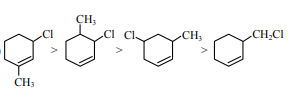2.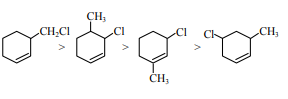3.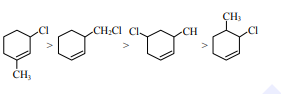4.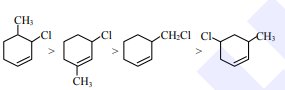Correct Option: 1

Solution:

As it is example of $\mathrm{SN}^{1}$.

so carbocation stability $\uparrow$, reaction rate $\uparrow$